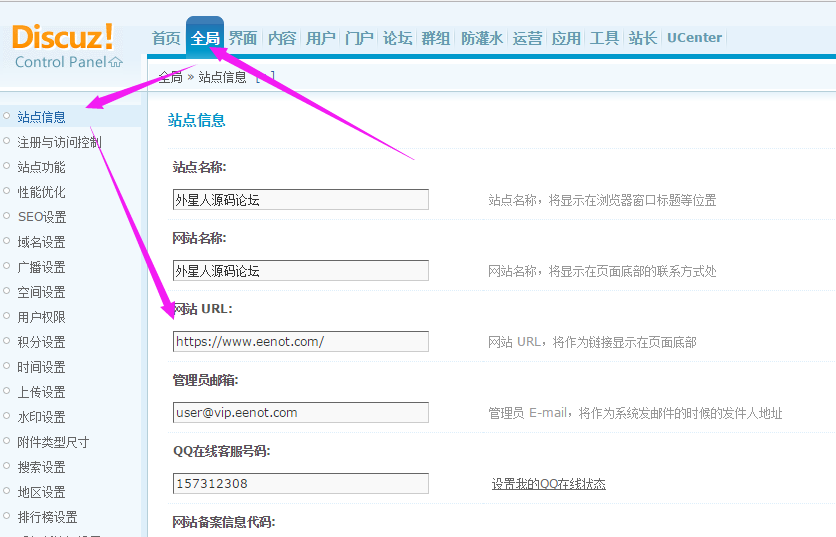Discuz!x 3.2全站切换到 HTTPS的修改方法``````\$_G['siteurl'] = dhtmlspecialchars('http'.(\$_G['isHTTPS'] ? 's' : '').'://'.\$_SERVER['HTTP_HOST'].\$sitepath.'/');
``````

``````\$_G['isHTTPS'] = true;
``````

``````define('UC_API', strtolower((\$_SERVER['HTTPS'] == 'on' ? 'https' : 'http').'://'.\$_SERVER['HTTP_HOST'].substr(\$_SERVER['PHP_SELF'], 0, strrpos(\$_SERVER['PHP_SELF'], '/'))));
``````

``````define('UC_API', strtolower('https'.'://'.\$_SERVER['HTTP_HOST'].substr(\$_SERVER['PHP_SELF'], 0, strrpos(\$_SERVER['PHP_SELF'], '/'))));
``````

``````http://discuz.gtimg.cn/cloud/scripts/discuz_tips.js
``````

``````https://discuz.gtimg.cn/cloud/scripts/discuz_tips.js
``````

``````\$comment[avatar]
``````

``````<!--{eval echo str_replace("http","https",\$comment[avatar]);}-->
``````